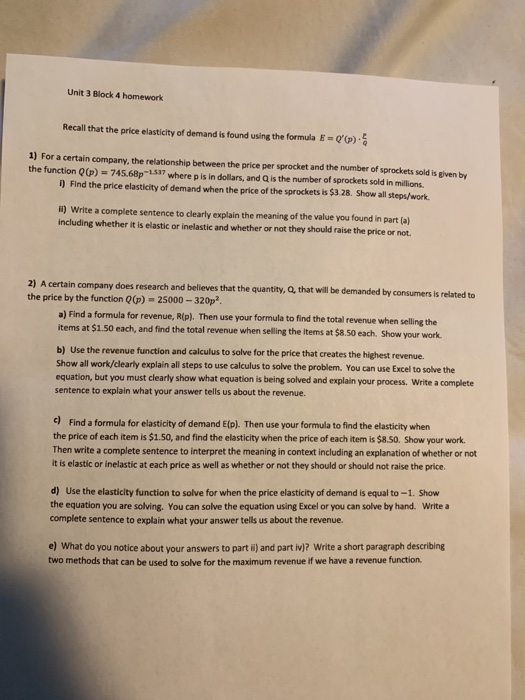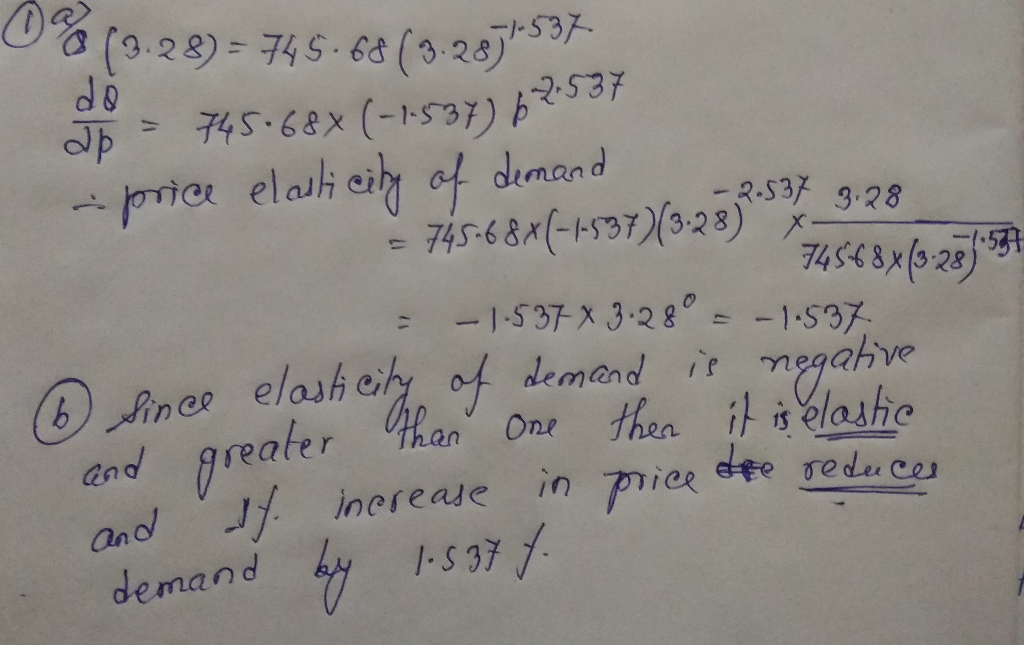# Unit 3 Block 4 homework Recall that the price elasticity of demand is found using the formula E =...##### Add Answer of: Unit 3 Block 4 homework Recall that the price elasticity of demand is found using the formula E =...
More Homework Help Questions Additional questions in this topic.

• #### The price elasticity of demand for senior citizens purchasing coffee from McDonald's is -5 while non senior citizens have a price elasticity of demand equal to -1.25

Need Online Homework Help?